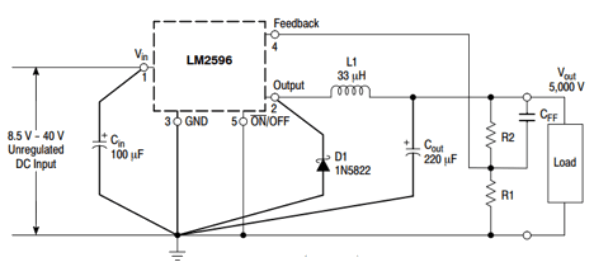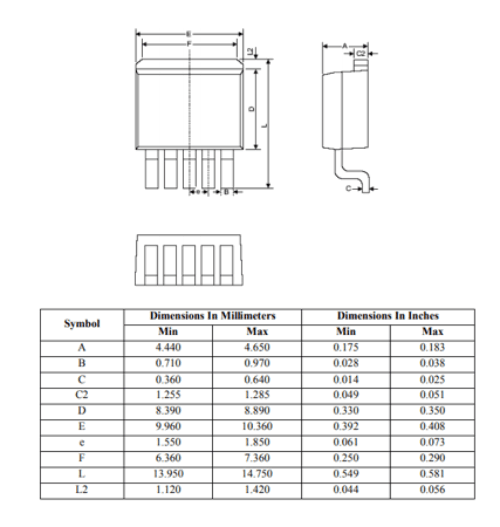# LM2596 3A Step Down Voltage Switching Regulator

[Click the image to enlarge it]

The LM2596 is a commonly used popular step-down switching regulator IC. The adjustable version can take in input voltage from 4.5V to 40V and convert it to variable voltage sourcing upto of 3A of continues current. Because of its high current capability is commonly used in power modules to power/control heavy loads.

### Pin Description

 Pin Number Pin Name Description 1 V-IN Input voltage that is to be regulated 2 V-OUT Stepped down Regulated output Voltage 3 Ground Connected to system ground 4 Feedback Sets the output voltage using divider network using output voltage feedback 5 ON/OFF Enable pin, should be grounded for normal operation

### Features

• 3A Step Down Voltage Regulator IC
• Available as 3.3V regulator, 5V Regulator, 12V Regulator and Variable regulator
• Input Supply Voltage: 4.5V to 40V
• Minimum Output Voltage: 3.16V
• Continuous Output Current: 3A
• Peak Output Current: 6.9A
• Switching Frequency: 150KHz
• Available in To-220 and To-263 Package

Note: The LM2596 is of many types. The adjustable version LM2596-ADJ is the most commonly used and hence we will discuss only about that in this article. The Complete Technical Details can be found at the datasheet given at the end of this page.

### Equivalent for LM2596

LM2576, BD9876, ACT4523

### Alternative Switching Regulators

XL6009, TPS5430, MC34063

### LM2596 Introduction

The LM2596 is known for its high current rating of 3A. It is available in many versions with fixed output voltage like 3.3V, 5V and 12V. But, the most famous one is the LM2596-ADJ which has variable output voltage. The IC is basically a buck converter that operates on 150KHz switching frequency, it takes in an input voltage and uses the internal switching circuit to regulated a desired output voltage. It has high efficiency and in-built Thermal shutdown and current limit functionalities. So if you are looking for a small high package, easy to use high current buck converter IC then LM2596 might me the right choice for you.

### How to use the LM2596 Regulator

The LM2596 is very easy to use because it requires very minimum number of components. The unregulated voltage is given to pin 1 (Vin) across a filter capacitor to reduce input noise. The ON/OFF or enable pin (pin 5) should be connected to ground to enable the IC. If made high the IC will enter shutdown mode and prevent leakage current. This feature will be useful to save input power when operating via battery. The feedback pin is the important pin which sets the output voltage. It sense the output voltage and based on the value of this output voltage the switching frequency of the internal switch is adjusted to provide the desired output voltage. Finally the output voltage is obtained through pin 2 through an LC filter. The complete circuit diagram is given below, you can often find these circuit in the LM2596 DC Converter module.### Calculating output voltage for LM2596

As told earlier the output voltage of the LM2596-ADJ can be controlled by using the feedback pin. The aboce circuit diagram shows the feedback pin getting the feedback voltage from a voltage divider circuit formed with Resistors R1 and R2. The value of this R1 and R2 decides the output voltage of the IC. The formula to calculate R1 and R2 is given below.

Vout = Vref (1.0 + R2/R1)

Here the value of Vref can be considered as 1.23V hence the formula becomes Vout = 1.23*(1+(R2/R1)), where the value of R1 should be between 1k to 5k. It is also possible to use a variable resistor in place of R1 to control the output voltage. This makes the IC easier to use in Variable power supply circuits.

### Applications

• Used in battery circuits since they have high efficiency
• Step-down Linear regulators
• Used in small SMPS circuits
• Battery Operated Applications
• Variable Voltage generators
• Miniature RPS Circuits

### 2D-ModelComponent Datasheet